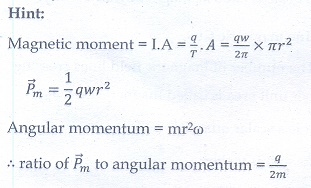# Unit III: Magnetism and magnetic effects of electric current - Online Test

Q1. The magnetic field at the center O of the following current loop isExplaination / Solution:Q2. An electron moves straight inside a charged parallel plate capacitor of uniform charge density σ. The time taken by the electron to cross the parallel plate capacitor when the plates of the capacitor are kept under constant magnetic field of inductionisExplaination / Solution: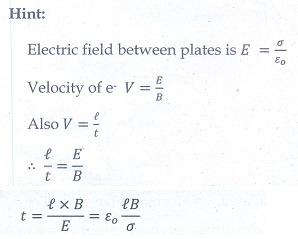Q3. The force experienced by a particle having mass m and charge q accelerated through a potential difference V when it is kept under perpendicular magnetic fieldis
Explaination / Solution:Q4. A circular coil of radius 5 cm and 50 turns carries a current of 3 ampere. The magnetic dipole moment of the coil is
Explaination / Solution: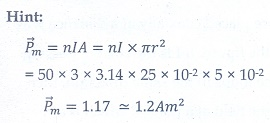Q5. A thin insulated wire forms a plane spiral of N = 100 tight turns carrying a current I = 8 m A (milli ampere). The radii of inside and outside turns are a = 50 mm and b = 100 mm respectively. The magnetic induction at the center of the spiral is
Explaination / Solution:Q6. Three wires of equal lengths are bent in the form of loops. One of the loops is circle, another is a semi-circle and the third one is a square. They are placed in a uniform magnetic field and same electric current is passed through them. Which of the following loop configuration will experience greater torque ?
Explaination / Solution: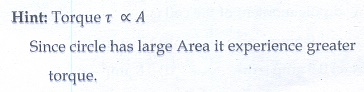Q7. Two identical coils, each with N turns and radius R are placed coaxially at a distance R as shown in the figure. If I is the current passing through the loops in the same direction, then the magnetic field at a point P which is at exactly at R/2 distance between two coils is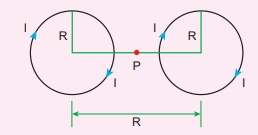Explaination / Solution: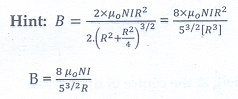Q8.

A wire of length l carries a current I along the Y direction and magnetic field is given byThe magnitude of Lorentz force acting on the wire is

Explaination / Solution: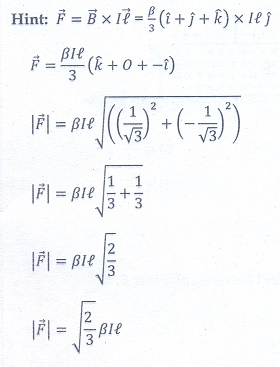Q9. A bar magnet of length l and magnetic moment M is bent in the form of an arc as shown in figure. The new magnetic dipole moment will be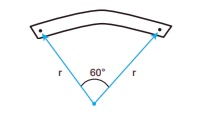Explaination / Solution: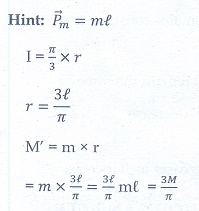Q10. A non-conducting charged ring of charge q, mass m and radius r is rotated with constant angular speed ω. Find the ratio of its magnetic moment with angular momentum is
Explaination / Solution: